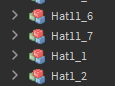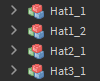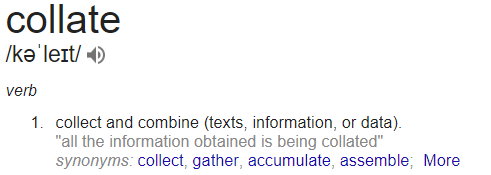# Behavior of the Explorer's sort, and table.sort()

table.sort
As a Roblox developer, it is currently inefficient to sort a table alphabetically (using table.sort(t) as intended). It results in an illogical manner. This is also occurs in the Explorer. It is intended to sort all objects in it alphabetically, including numbers.
However because of how it’s handled, things like this happen:Which should beIt would make much more sense if this code:

``````local t = {"1","5","3","4","7","2","6","10","8","9"}
table.sort(t)
for _ , number in pairs (t) do
print(number)
end
``````

Would result in:

``````>1
>2
>3
>4
>5
>6
>7
>8
>9
>10
``````
3 Likes

But that’s how a proper alphabetical sort works. What you’re asking for is a more contextual-based sort. Windows started doing this in either Vista or Windows 7, but before had the same issue. (e.g. a file named “Image_10” would show before “Image_2”).

In an alphabetical sort, the algorithm looks at a character-per-character basis; it doesn’t consider the whole context (e.g. finding a multi-digit number).

Changing the default behavior of `table.sort` could result in game-breaking issues.

4 Likes

Pro tip: Add a 0 before the number, if you plan them to have double digits, or tripple digits.

For some reason they always read from left to right order of characters.

4 Likes

I agree with this. If you’re generating things progamatically, you can automatically pad your numbers using this format:

``````string.format("%.3i", number)
``````

Where the “3” represents the number of digits. For instance:

``````for i = 1,100 do print(string.format("Hello_%.3i", i)) end
``````

Output:

``````Hello_001
Hello_002
Hello_003
...
Hello_098
Hello_099
Hello_100
``````
8 Likes

I did just test this, and worked as you showed. Can I ask what the two i 's represents in

``````string.format("Hello_%.3i", i)
``````

In the `format` function, an `i` or `d` represent an integer. The `i` by itself as the 2nd parameter is from the `for` loop. Check out the Format String page for more info.

I did some more research on this. Apparently what you’re looking for is something called a “Natural Sort”. After some googling, I found a Lua implementation. I reformatted it just a bit:

[Code Source]

``````function NaturalSort(tbl)
local function Convert(s)
local res, dot = "", ""
for n, m, c in tostring(s):gmatch("(0*(%d*))(.?)") do
if (n == "") then
dot, c = "", dot .. c
else
res = res .. (dot == "" and ("%03d%s"):format(#m, m) or "." .. n)
dot, c = c:match("(%.?)(.*)")
end
res = res .. c:gsub(".", "\0%0")
end
return res
end
table.sort(tbl, function(a, b)
local ca, cb = Convert(a), Convert(b)
return (ca < cb or ca == cb and a < b)
end)
return tbl
end
``````

Example:

``````local strs = {"Hi10", "Hi2", "Hi1", "Hi32", "Hi5", "Hi3", "Hi0"}
NaturalSort(strs)
for i,s in pairs(strs) do print(i,s) end
``````

And here is the blog post that gave me lots of good info on this subject.

3 Likes

Here’s what I went with. It works by continually splitting each string by its first sequence of digits.

``````local function NaturalSort(a, b)
-- Split string by first sequence of digits.
local function findNum(s)
local i, j, n = s:find("(%d+)")
if not i then
return s, 0, ""
end
-- Return prefix, number, suffix
return s:sub(1, i-1), tonumber(n), s:sub(j+1)
end

local apfx, anum, bpfx, bnum
while true do
if b == "" then
return false -- b < a
end
if a == "" then
return true -- a < b
end
apfx, anum, a = findNum(a)
bpfx, bnum, b = findNum(b)
if apfx ~= bpfx then
return apfx < bpfx
end
if anum ~= bnum then
return anum < bnum
end
end
end

local t = {"foo10","bar29","foo11","bar2","foo1","foo7","bar25","foo12","foo9"}
table.sort(t, NaturalSort)
for i = 1,#t do print(t[i]) end
``````

Also, the concept of sort order seems to be referred to as “collation”, if you want to look up more.

2 Likes

@Anaminus & @Crazyman32
Thank you for the help in this. I’ll be looking further into this. I really appreciate it